Get instant live expert help with Excel or Google Sheets“My Excelchat expert helped me in less than 20 minutes, saving me what would have been 5 hours of work!”

#### Post your problem and you’ll get Expert help in seconds.

Your message must be at least 40 characters
Our professional Expert are available now. Your privacy is guaranteed.

# Excel MEDIAN Function

The MEDIAN function is one of the measures of central tendency in Excel.  The median of a set of numbers is defined as the middle number.  This tutorial will assist all levels of Excel users in using the MEDIAN function.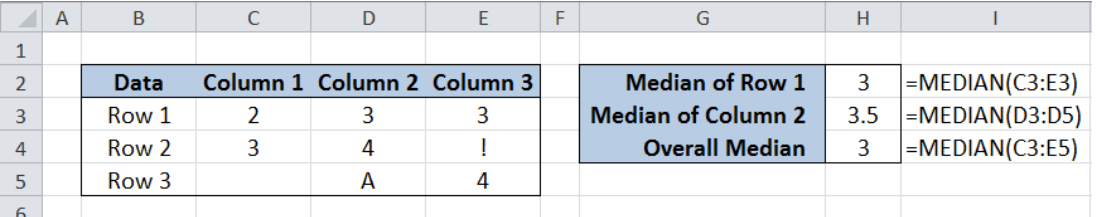Figure 1. Final result of the MEDIAN function

## Syntax of the MEDIAN Function

Returns the median of the given numbers

### Syntax:

`=MEDIAN(number1, [number2], ...)`

• Number1, number2 – any number, reference to a number, or array that contains numbers; only number1 is required while the succeeding numbers are optional

## Setting up our Data

Our data consists of three rows and three columns.  Note that cell C5 is blank, cell D5 contains a letter “A” and cell E4 contains the character “!”.  In column H, we want to determine the median of Row 1, Column 2 and the Overall median.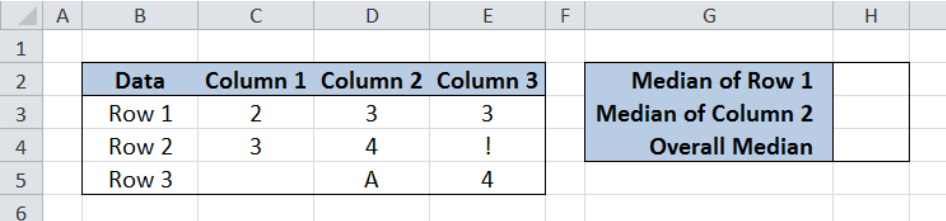Figure 2. Sample data for calculating the median

## Calculate the Median

### Median of Row 1

The parameter for the median function could be any number or range of cells containing numbers.  To determine the median of the 1st row, follow these steps:

Step 1. Select H2

Step 2.  Enter the formula: `=MEDIAN(C3:E3)`

Step 2. Press ENTER

The result in cell H2 is the median 3, which means that half of the numbers in row 1 have values below 3, and half the numbers have values above 3.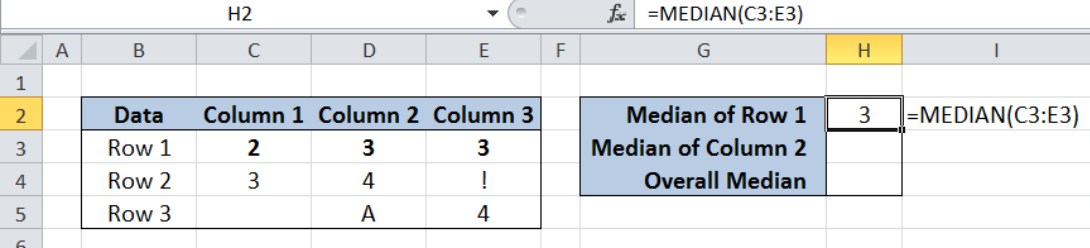Figure 3. Calculating the median of row 1

### Median of Column 2

To determine the median of the 2nd column, follow these steps:

Step 1. Select H3

Step 2.  Enter the formula: `=MEDIAN(D3:D5)`

Step 2. Press ENTER

The result in cell H3 is the median 3.5.  Note that the MEDIAN function ignores cells that contain text (cell D5).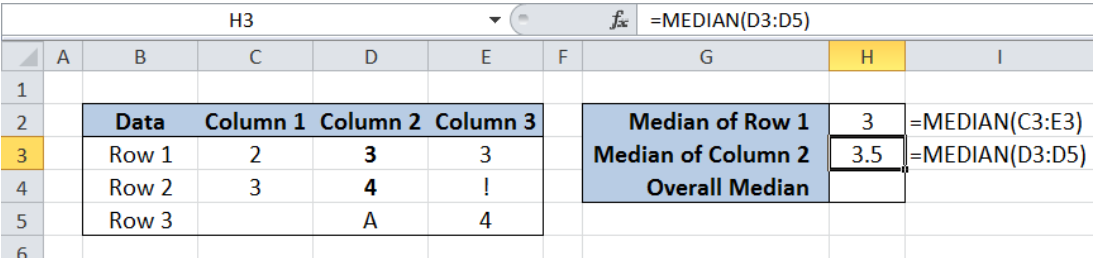Figure 4.  Calculating the median of column 2

### Overall Median

To determine the overall median of our data set, follow these steps:

Step 1. Select H4

Step 2.  Enter the formula: `=MEDIAN(C3:E5)`

Step 2. Press ENTER

The result in cell H4 is the median 3.  Note that the MEDIAN function ignores cells that have values other than numbers (cells D5 and E4), and cells that are empty (cell C5).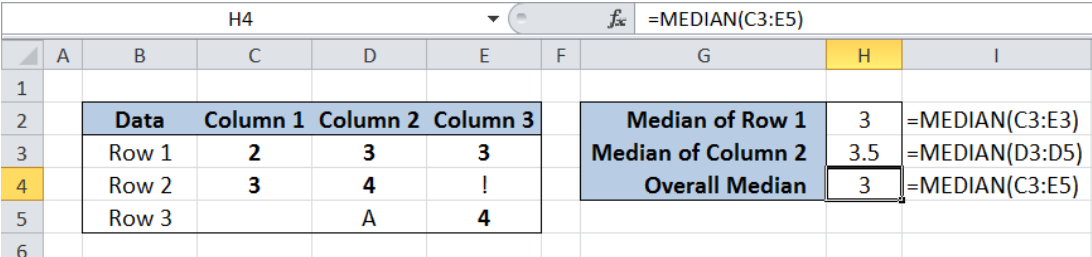Figure 5.  Calculating the overall median

## Notes

• When the total number of arguments is odd, the MEDIAN function determines the median as the middle number in the group.
• When the total number of arguments is even,  the MEDIAN function calculates the median as the average of the two numbers in the middle.
• MEDIAN returns an error when there are cells containing error values or values that cannot be converted into numbers
• The MEDIAN function ignores cells that contain text, logical values, or cells that are empty

Most of the time, the problem you will need to solve will be more complex than a simple application of a formula or function. If you want to save hours of research and frustration, try our live Excelchat service! Our Excel Experts are available 24/7 to answer any Excel question you may have. We guarantee a connection within 30 seconds and a customized solution within 20 minutes.

### Did this post not answer your question? Get a solution from connecting with the expert.Another blog reader asked this question today on Excelchat:
Solution examplesNeed to calculate out two equally spaced points between a range of dates. Basically like the MEDIAN function between two cells that have dates, but I need to produce two equally spaced points between that range. For example. If I have the following date range (A1) 2/2/2018 (B1) 7/2/2018, then that's roughly 150 days total. I want 4 equally spaced points (Start, Start-Mid, Mid-End, End). So I'd say that's 50 day chunks spaced euqally. Which would produce 4 total dates 2/2/2018 - 3/24/2018 - 5/13/2018 - 7/2/2018.
Solved by K. J. in 20 minsi have this formula currently which works but not if I want it for every day - any ideas ?? (NETWORKDAYS.INTL(L1362,M1362,11,BH!A:A)-1)*("17:30"-"8:30")+IF(NETWORKDAYS.INTL(M1362,M1362,11,BH!A:A),MEDIAN(MOD(M1362,1),"8:30","17:30"),"17:30")-MEDIAN(NETWORKDAYS.INTL(L1362,L1362,11,BH!A:A)*MOD(L1362,1),"8:30","17:30")
Solved by A. D. in 28 mins## Subscribe to Excelchat.coAnother blog reader asked this question today on Excelchat: